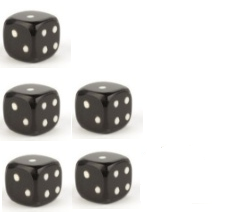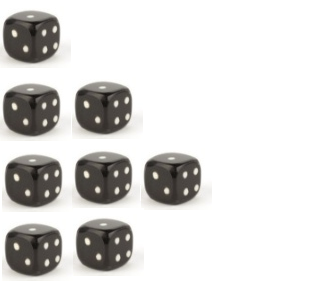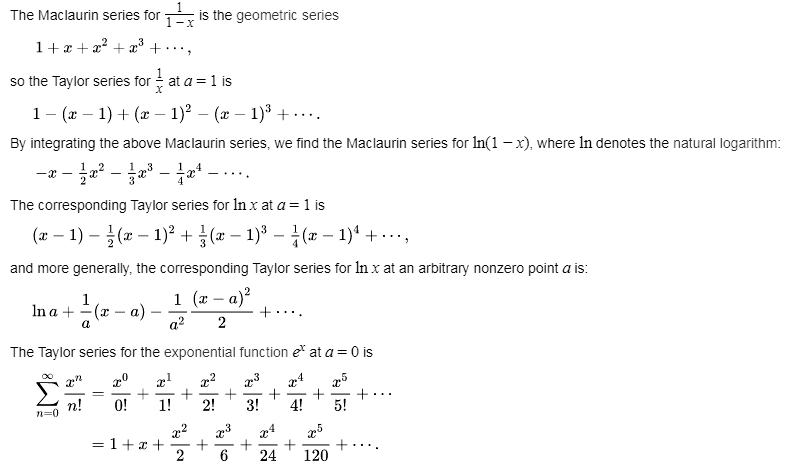﻿ C programming exercises: Math - w3resource# C programming Math : Exercises, Practice and Solution

## C programming Mathematics [28 exercises with solution]

[An editor is available at the bottom of the page to write and execute the scripts.]

1. Write a C program to reverse the digits of a given integer. Go to the editor
Example:
Input:
i = 123
i = 208478933
i = -73634
Output:
Reverse integer: 321
Reverse integer: 339874802
Reverse integer: -43637
Click me to see the solution

2. Write a C program to check whether an integer is a palindrome or not. An integer is a palindrome when it reads the same forward as backward. Go to the editor
Example:
Input:
i = 1221
i = -121
i = 100
Output:
Is Palindrome: 1
Is Palindrome: 0
Is Palindrome: 0
Click me to see the solution

3. Write a C program to divide two integers (dividend and divisor) without using multiplication, division and mod operator. Go to the editor
Example:
Input:
dividend_num = 7
divisor_num = 2

dividend_num = -17
divisor_num = 5

dividend_num = 35
divisor_num = 7
Output:
Result: 3
Result: -3
Result: 5
Click me to see the solution

4. Write a C program to calculate x raised to the power n (xn). Go to the editor
Example:
Input:
x = 7.0
n = 2

x = 6.2
n = 3
Output:
Result:(x^n) : 49.000000
Result:(x^n) : 238.328000
Click me to see the solution

5. The following set contains a total of n! unique permutations
Set: [1, 2, 3, ..., n]
If n =3 we will get the following sequence:
1. "123"
2. "132"
3. "213"
4. "231"
5. "312"
6. "321"
Input: n = 3, k = 4
Output: "231"
Write a C program to get the kth permutation sequence from two given integers n and k where n is between 1 and 9 inclusive and k is between 1 and n! inclusive. Go to the editor
Example:
Input:
n = 3
int k = 2

n = 4
k = 7
Output:
Kth sequence: 132
Kth sequence: 2134
Click me to see the solution

6. Write a C program to check if a given string can be interpreted as a decimal number. Go to the editor
Example:
Input:
str_num1[ ] ="1234"
str_num2[ ]=" 0.1 "
str_num3[ ]=" -90e3 "
str_num4[ ]=" 99e2.5 "
Output:
Is the above string is a number? 1
Is the above string is a number? 1
Is the above string is a number? 1
Is the above string is a number? 0
Click me to see the solution

7. Write a C program to get the fraction part from two given integers representing the numerator and denominator in string format. Go to the editor
Example:
Input:
n = 3
d = 2

n = 4
d = 7
Output:
Fractional part: 1.5
Fractional part: 0.(571428)
Click me to see the solution

8. Write a C program to get the Excel column title that corresponds to a given column number (integer value). Go to the editor
For example:
1 -> A
2 -> B
3 -> C
...
26 -> Z
27 -> AA
28 -> AB
...
Example:
Input:
n = 3
n = 27
n = 151
Output:
Excel column title: C
Excel column title: AA
Excel column title: EU
Click me to see the solution

9. Write a C program to get the column number (integer value) that corresponds to a column title as appear in an Excel sheet. Go to the editor
For example:
A -> 1
B -> 2
C -> 3
...
Z -> 26
AA -> 27
AB -> 28
...
Example:
Input:
col_title1[ ] ="C"
col_title2[ ] ="AC"
col_title3[ ] ="ZY"
Output:
Corresponding number: 3
Corresponding number: 29
Corresponding number: 701
Click me to see the solution

10. Write a C program to find the number of trailing zeroes in a given factorial. Go to the editor
Example 1:
Input: 4
Output: 0
Explanation: 4! = 24, no trailing zero.
Example 2:
Input: 6
Output: 1
Explanation: 6! = 720, one trailing zero.

Example:
Input:
n = 4
n = 5
Output:
Number of trailing zeroes of factorial 4 is 0
Number of trailing zeroes of factorial 5 is 1
Click me to see the solution

11. Write a C program to count the total number of digit 1 appearing in all positive integers less than or equal to a given integer n. Go to the editor
Example:
Input n = 12,
Return 5, because digit 1 occurred 5 times in the following numbers: 1, 10, 11, 12.
Example:
Input:
n = 12
n = 30
Output:
Total number of digit 1 appearing in 12 (less than or equal) is 5.
Total number of digit 1 appearing in 30 (less than or equal) is 13.
Click me to see the solution

12. Write a C programming to add repeatedly all digits of a given non-negative number until the result has only one digit. Go to the editor
Example:
Input: 48
Output: 2
Explanation: The formula is like: 4 + 8 = 12, 1 + 2 = 3.
Click me to see the solution

13. Write a C programming to check if a given integer is a power of three. Go to the editor
Example:
Input: 9
Output: true
Input: 81
Output: true
Input: 45
Output: false
Click me to see the solution

14. For a non negative integer in the range 0 ≤ i ≤ n write a C programming to calculate the number of 1's in their binary representation and return them as an array. Go to the editor
Example:
Input:
Number: 7
Number of 1's in the binary representation:
0: 0
1: 1
2: 1
3: 2
4: 1
5: 2
Click me to see the solution

15. Write a C programming to get the maximum product from a given integer after breaking the integer into the sum of at least two positive integers. Go to the editor
Example:
Input: 12
Output: 81
Explanation: 12 = 3 + 3 + 3 + 3, 3 x 3 × 3 × 3 = 81.
Input: 7
Output: 12
Explanation: 7 = 3 + 2 + 2, 3 x 2 x 2 = 12.
Click me to see the solution

16. Lexicographical order:
From Wikipedia,
In mathematics, the lexicographic or lexicographical order (also known as lexical order, dictionary order, alphabetical order or lexicographic(al) product) is a generalization of the way words are alphabetically ordered based on the alphabetical order of their component letters. This generalization consists primarily in defining a total order on the sequences (often called strings in computer science) of elements of a finite totally ordered set, often called an alphabet.
Write a C programming to print numbers from 1 to an given integer(N) in lexicographic order. Go to the editor
Example:
Input: 10
Output:
Print numbers from 1 to 10 in lexicographic order-
1 10 2 3 4 5 6 7 8 9
Input: 25
Output:
Print numbers from 1 to 25 in lexicographic order-
1 10 11 12 13 14 15 16 17 18 19 2 20 21 22 23 24 25 3 4 5 6 7 8 9
Click me to see the solution

17. Write a C programming to find the nth digit of number 1 to n?. Go to the editor
Infinite integer sequence: 1, 2, 3, 4, 5, 6, 7, 8, 9, 10, 11, 12 .. where n is a positive integer.
Example:
Input:
7
Output:
7
Input:
12
Output:
1
The 12th digit of the sequence 1, 2, 3, 4, 5, 6, 7, 8, 9, 10, 11, ... is 1, which is part of the number 11.
Click me to see the solution

18. Write a C programming to find the total number of full staircase rows that can be formed from given number of dices. Go to the editor
Example 1:
n = 5
The dices can form the following rows:As the 3rd row is incomplete the program will return 2 (full staircase rows).
Example 1:
n = 8 The dices can form the following rows:As the 4th row is incomplete the program will return 3 (full staircase rows).
Click me to see the solution

19. Write a C program to find the square root of a number using Babylonian method. Go to the editor
Example 1:
Input: n = 50
Output: 7.071068
Example 2:
Input: n = 17
Output: 4.123106
Click me to see the solution

20. Write a C program to multiply two integers without using multiplication, division, bitwise operators, and loops. Go to the editor
Example 1:
Input: n1 = 50
Input: n2 = 12
Output: 600
Example 2:
Input: n1 = 0
Input: n2 = 12
Output: 0
Click me to see the solution

21. Write a C program to calculate and print average (or mean) of the stream of given numbers. Go to the editor
Example 1:
Input:
arr[] = {10, 20, 30, 40, 50, 60, 70, 80, 90, 100}
Output:
Average of 1 numbers is 10.000000
Average of 2 numbers is 15.000000
Average of 3 numbers is 20.000000
Average of 4 numbers is 25.000000
Average of 5 numbers is 30.000000
Average of 6 numbers is 35.000000
Average of 7 numbers is 40.000000
Average of 8 numbers is 45.000000
Average of 9 numbers is 50.000000
Average of 10 numbers is 55.000000
Click me to see the solution

22. Write a C program to count the numbers without digit 7, from 1 to a given number. Go to the editor
Example 1:
Input: n = 10
Output: 9
Example 2:
Input: n = 687
Output: 555
Click me to see the solution

23. Write a C program to find next smallest palindrome of a given number. Go to the editor
From Wikipedia,
A palindrome is a word, number, phrase, or other sequence of characters which reads the same backward as forward, such as madam, racecar. There are also numeric palindromes, including date/time stamps using short digits 11/11/11 11:11 and long digits 02/02/2020. Sentence-length palindromes may be written when allowances are made for adjustments to capital letters, punctuation, and word dividers, such as "A man, a plan, a canal, Panama!".
Example 1:
Input: n = 121
Output: Next smallest palindrome of 121 is 131
Click me to see the solution

24. Write a C program to calculate e raise to the power x using sum of first n terms of Taylor Series. Go to the editor
From Wikipedia,
In mathematics, a Taylor series is a representation of a function as an infinite sum of terms that are calculated from the values of the function's derivatives at a single point.
Example:
The Taylor series for any polynomial is the polynomial itself.The above expansion holds because the derivative of ex with respect to x is also ex, and e0 equals 1.
This leaves the terms (x − 0)n in the numerator and n! in the denominator for each term in the infinite sum.
Example 1:
Input: n = 25
float x= 5.0
Output: e^x = 148.413162
Click me to see the solution

25. Write a C program to print all prime factors of a given number. Go to the editor
Example 1:
Input: n = 75
Output: All prime factors of 75 are: 3 5 5
Click me to see the solution

26. Write a C program to check if a given number is Fibonacci number or not. Go to the editor
In mathematics, the Fibonacci numbers, commonly denoted Fn form a sequence, called the Fibonacci sequence, such that each number is the sum of the two preceding ones, starting from 0 and 1. That is, and for n > 1. By starting with 1 and 2, the first 10 terms will be: 1, 2, 3, 5, 8, 13, 21, 34, 55, 89.
Example 1:
Input: n = 8
Output: 1
Click me to see the solution

27. Write a C program to multiply two numbers using bitwise operators. Go to the editor

Example 1:
Input: int x = 8
int y = 9
Output: Product of 8 and 9 using bitwise operators is: 72
Click me to see the solution

28. Write a C program to find angle between given hour and minute hands. Go to the editor

Example 1:
Input: int ha = 11
int ma = 30 Output: Angle between hour and minute hands 165
Click me to see the solution

C Programming Code Editor:

More to Come !

Do not submit any solution of the above exercises at here, if you want to contribute go to the appropriate exercise page.

﻿

## C Programming: Tips of the Day

The tilde operator in C:

The ~ operator is bitwise NOT, it inverts the bits in a binary number:

```NOT 011100
= 100011
```

Ref : https://bit.ly/3DR1N24